# Group Sparse inverse covariance for multi-subject connectome#

This example shows how to estimate a connectome on a group of subjects using the group sparse inverse covariance estimate.

Note

If you are using Nilearn with a version older than `0.9.0`, then you should either upgrade your version or import maskers from the `input_data` module instead of the `maskers` module.

That is, you should manually replace in the following example all occurrences of:

```from nilearn.maskers import NiftiMasker
```

with:

```from nilearn.input_data import NiftiMasker
```
```import numpy as np
from nilearn import plotting

n_subjects = 4  # subjects to consider for group-sparse covariance (max: 40)

def plot_matrices(cov, prec, title, labels):
"""Plot covariance and precision matrices, for a given processing."""
prec = prec.copy()  # avoid side effects

# Put zeros on the diagonal, for graph clarity.
size = prec.shape
prec[list(range(size)), list(range(size))] = 0
span = max(abs(prec.min()), abs(prec.max()))

# Display covariance matrix
plotting.plot_matrix(
cov,
cmap=plotting.cm.bwr,
vmin=-1,
vmax=1,
title=f"{title} / covariance",
labels=labels,
)
# Display precision matrix
plotting.plot_matrix(
prec,
cmap=plotting.cm.bwr,
vmin=-span,
vmax=span,
title=f"{title} / precision",
labels=labels,
)
```

## Fetching datasets#

```from nilearn import datasets

msdl_atlas_dataset = datasets.fetch_atlas_msdl()
rest_dataset = datasets.fetch_development_fmri(n_subjects=n_subjects)

# print basic information on the dataset
print(
f"First subject functional nifti image (4D) is at: {rest_dataset.func}"
)
```
```First subject functional nifti image (4D) is at: /home/yasmin/nilearn/nilearn_data/development_fmri/development_fmri/sub-pixar123_task-pixar_space-MNI152NLin2009cAsym_desc-preproc_bold.nii.gz
```

## Extracting region signals#

```from nilearn.maskers import NiftiMapsMasker

msdl_atlas_dataset.maps,
resampling_target="maps",
detrend=True,
high_variance_confounds=True,
low_pass=None,
high_pass=0.01,
t_r=2,
standardize="zscore_sample",
memory="nilearn_cache",
memory_level=1,
verbose=2,
)

subject_time_series = []
func_filenames = rest_dataset.func
confound_filenames = rest_dataset.confounds
for func_filename, confound_filename in zip(
func_filenames, confound_filenames
):
print(f"Processing file {func_filename}")

subject_time_series.append(region_ts)
```
```[NiftiMapsMasker.fit] loading regions from /home/yasmin/nilearn/nilearn_data/msdl_atlas/MSDL_rois/msdl_rois.nii
________________________________________________________________________________
[Memory] Calling nilearn.image.image.high_variance_confounds...
__________________________________________high_variance_confounds - 1.0s, 0.0min
________________________________________________________________________________
'clean_kwargs': {},
'detrend': True,
'dtype': None,
'high_pass': 0.01,
'high_variance_confounds': True,
'low_pass': None,
'maps_img': '/home/yasmin/nilearn/nilearn_data/msdl_atlas/MSDL_rois/msdl_rois.nii',
'reports': True,
'smoothing_fwhm': None,
'standardize': 'zscore_sample',
'standardize_confounds': True,
't_r': 2,
'target_affine': array([[   4.,    0.,    0.,  -78.],
[   0.,    4.,    0., -111.],
[   0.,    0.,    4.,  -51.],
[   0.,    0.,    0.,    1.]]),
'target_shape': (40, 48, 35)}, confounds=[ array([[-0.174325, ..., -0.048779],
...,
[-0.044073, ...,  0.155444]]),
/home/yasmin/miniconda3/envs/nilearn-dev/lib/python3.10/site-packages/joblib/memory.py:349: FutureWarning:

The default strategy for standardize is currently 'zscore' which incorrectly uses population std to calculate sample zscores. The new strategy 'zscore_sample' corrects this behavior by using the sample std. In release 0.13, the default strategy will be replaced by the new strategy and the 'zscore' option will be removed. Please use 'zscore_sample' instead.

_______________________________________________filter_and_extract - 6.7s, 0.1min
________________________________________________________________________________
[Memory] Calling nilearn.image.image.high_variance_confounds...
__________________________________________high_variance_confounds - 0.9s, 0.0min
________________________________________________________________________________
'clean_kwargs': {},
'detrend': True,
'dtype': None,
'high_pass': 0.01,
'high_variance_confounds': True,
'low_pass': None,
'maps_img': '/home/yasmin/nilearn/nilearn_data/msdl_atlas/MSDL_rois/msdl_rois.nii',
'reports': True,
'smoothing_fwhm': None,
'standardize': 'zscore_sample',
'standardize_confounds': True,
't_r': 2,
'target_affine': array([[   4.,    0.,    0.,  -78.],
[   0.,    4.,    0., -111.],
[   0.,    0.,    4.,  -51.],
[   0.,    0.,    0.,    1.]]),
'target_shape': (40, 48, 35)}, confounds=[ array([[-0.151677, ..., -0.057023],
...,
[-0.206928, ...,  0.102714]]),
/home/yasmin/miniconda3/envs/nilearn-dev/lib/python3.10/site-packages/joblib/memory.py:349: FutureWarning:

The default strategy for standardize is currently 'zscore' which incorrectly uses population std to calculate sample zscores. The new strategy 'zscore_sample' corrects this behavior by using the sample std. In release 0.13, the default strategy will be replaced by the new strategy and the 'zscore' option will be removed. Please use 'zscore_sample' instead.

_______________________________________________filter_and_extract - 6.7s, 0.1min
________________________________________________________________________________
[Memory] Calling nilearn.image.image.high_variance_confounds...
__________________________________________high_variance_confounds - 0.9s, 0.0min
________________________________________________________________________________
'clean_kwargs': {},
'detrend': True,
'dtype': None,
'high_pass': 0.01,
'high_variance_confounds': True,
'low_pass': None,
'maps_img': '/home/yasmin/nilearn/nilearn_data/msdl_atlas/MSDL_rois/msdl_rois.nii',
'reports': True,
'smoothing_fwhm': None,
'standardize': 'zscore_sample',
'standardize_confounds': True,
't_r': 2,
'target_affine': array([[   4.,    0.,    0.,  -78.],
[   0.,    4.,    0., -111.],
[   0.,    0.,    4.,  -51.],
[   0.,    0.,    0.,    1.]]),
'target_shape': (40, 48, 35)}, confounds=[ array([[ 0.127944, ..., -0.087084],
...,
[-0.015679, ..., -0.02587 ]]),
/home/yasmin/miniconda3/envs/nilearn-dev/lib/python3.10/site-packages/joblib/memory.py:349: FutureWarning:

The default strategy for standardize is currently 'zscore' which incorrectly uses population std to calculate sample zscores. The new strategy 'zscore_sample' corrects this behavior by using the sample std. In release 0.13, the default strategy will be replaced by the new strategy and the 'zscore' option will be removed. Please use 'zscore_sample' instead.

_______________________________________________filter_and_extract - 6.5s, 0.1min
________________________________________________________________________________
[Memory] Calling nilearn.image.image.high_variance_confounds...
__________________________________________high_variance_confounds - 0.9s, 0.0min
________________________________________________________________________________
'clean_kwargs': {},
'detrend': True,
'dtype': None,
'high_pass': 0.01,
'high_variance_confounds': True,
'low_pass': None,
'maps_img': '/home/yasmin/nilearn/nilearn_data/msdl_atlas/MSDL_rois/msdl_rois.nii',
'reports': True,
'smoothing_fwhm': None,
'standardize': 'zscore_sample',
'standardize_confounds': True,
't_r': 2,
'target_affine': array([[   4.,    0.,    0.,  -78.],
[   0.,    4.,    0., -111.],
[   0.,    0.,    4.,  -51.],
[   0.,    0.,    0.,    1.]]),
'target_shape': (40, 48, 35)}, confounds=[ array([[-0.089762, ..., -0.062316],
...,
[-0.065223, ..., -0.022868]]),
/home/yasmin/miniconda3/envs/nilearn-dev/lib/python3.10/site-packages/joblib/memory.py:349: FutureWarning:

The default strategy for standardize is currently 'zscore' which incorrectly uses population std to calculate sample zscores. The new strategy 'zscore_sample' corrects this behavior by using the sample std. In release 0.13, the default strategy will be replaced by the new strategy and the 'zscore' option will be removed. Please use 'zscore_sample' instead.

_______________________________________________filter_and_extract - 6.6s, 0.1min
```

## Computing group-sparse precision matrices#

```from nilearn.connectome import GroupSparseCovarianceCV

gsc = GroupSparseCovarianceCV(verbose=2)
gsc.fit(subject_time_series)

try:
from sklearn.covariance import GraphicalLassoCV
except ImportError:
# for Scitkit-Learn < v0.20.0
from sklearn.covariance import GraphLassoCV as GraphicalLassoCV

gl = GraphicalLassoCV(verbose=2)
gl.fit(np.concatenate(subject_time_series))
```
```[Parallel(n_jobs=1)]: Using backend SequentialBackend with 1 concurrent workers.
[GroupSparseCovarianceCV.fit] Log-likelihood on test set is decreasing. Stopping at iteration 2
[GroupSparseCovarianceCV.fit] Log-likelihood on test set is decreasing. Stopping at iteration 7
[GroupSparseCovarianceCV.fit] Log-likelihood on test set is decreasing. Stopping at iteration 0
[Parallel(n_jobs=1)]: Done   1 out of   1 | elapsed:    1.5s remaining:    0.0s
[GroupSparseCovarianceCV.fit] Log-likelihood on test set is decreasing. Stopping at iteration 2
[GroupSparseCovarianceCV.fit] Log-likelihood on test set is decreasing. Stopping at iteration 0
[GroupSparseCovarianceCV.fit] Log-likelihood on test set is decreasing. Stopping at iteration 2
[GroupSparseCovarianceCV.fit] Log-likelihood on test set is decreasing. Stopping at iteration 0
[GroupSparseCovarianceCV.fit] Log-likelihood on test set is decreasing. Stopping at iteration 1
[GroupSparseCovarianceCV.fit] Log-likelihood on test set is decreasing. Stopping at iteration 1
[GroupSparseCovarianceCV.fit] Log-likelihood on test set is decreasing. Stopping at iteration 0
[GroupSparseCovarianceCV.fit] Log-likelihood on test set is decreasing. Stopping at iteration 2
[GroupSparseCovarianceCV.fit] Log-likelihood on test set is decreasing. Stopping at iteration 6
[GroupSparseCovarianceCV.fit] Log-likelihood on test set is decreasing. Stopping at iteration 0
[Parallel(n_jobs=1)]: Done   5 out of   5 | elapsed:    7.9s finished
[GroupSparseCovarianceCV.fit] [GroupSparseCovarianceCV] Done refinement  0 out of 4
[Parallel(n_jobs=1)]: Using backend SequentialBackend with 1 concurrent workers.
[GroupSparseCovarianceCV.fit] Log-likelihood on test set is decreasing. Stopping at iteration 3
[GroupSparseCovarianceCV.fit] Log-likelihood on test set is decreasing. Stopping at iteration 6
[GroupSparseCovarianceCV.fit] Log-likelihood on test set is decreasing. Stopping at iteration 1
[GroupSparseCovarianceCV.fit] Log-likelihood on test set is decreasing. Stopping at iteration 0
[Parallel(n_jobs=1)]: Done   1 out of   1 | elapsed:    1.6s remaining:    0.0s
[GroupSparseCovarianceCV.fit] Log-likelihood on test set is decreasing. Stopping at iteration 4
[GroupSparseCovarianceCV.fit] Log-likelihood on test set is decreasing. Stopping at iteration 0
[GroupSparseCovarianceCV.fit] Log-likelihood on test set is decreasing. Stopping at iteration 3
[GroupSparseCovarianceCV.fit] Log-likelihood on test set is decreasing. Stopping at iteration 0
[GroupSparseCovarianceCV.fit] Log-likelihood on test set is decreasing. Stopping at iteration 0
[GroupSparseCovarianceCV.fit] Log-likelihood on test set is decreasing. Stopping at iteration 3
[GroupSparseCovarianceCV.fit] Log-likelihood on test set is decreasing. Stopping at iteration 6
[GroupSparseCovarianceCV.fit] Log-likelihood on test set is decreasing. Stopping at iteration 0
[Parallel(n_jobs=1)]: Done   5 out of   5 | elapsed:   13.2s finished
[GroupSparseCovarianceCV.fit] [GroupSparseCovarianceCV] Done refinement  1 out of 4
[Parallel(n_jobs=1)]: Using backend SequentialBackend with 1 concurrent workers.
[GroupSparseCovarianceCV.fit] Log-likelihood on test set is decreasing. Stopping at iteration 5
[GroupSparseCovarianceCV.fit] Log-likelihood on test set is decreasing. Stopping at iteration 1
[GroupSparseCovarianceCV.fit] Log-likelihood on test set is decreasing. Stopping at iteration 1
[Parallel(n_jobs=1)]: Done   1 out of   1 | elapsed:    2.1s remaining:    0.0s
[GroupSparseCovarianceCV.fit] Log-likelihood on test set is decreasing. Stopping at iteration 9
[GroupSparseCovarianceCV.fit] Log-likelihood on test set is decreasing. Stopping at iteration 10
[GroupSparseCovarianceCV.fit] Log-likelihood on test set is decreasing. Stopping at iteration 0
[GroupSparseCovarianceCV.fit] Log-likelihood on test set is decreasing. Stopping at iteration 5
[GroupSparseCovarianceCV.fit] Log-likelihood on test set is decreasing. Stopping at iteration 0
[Parallel(n_jobs=1)]: Done   5 out of   5 | elapsed:   15.3s finished
[GroupSparseCovarianceCV.fit] [GroupSparseCovarianceCV] Done refinement  2 out of 4
[Parallel(n_jobs=1)]: Using backend SequentialBackend with 1 concurrent workers.
[GroupSparseCovarianceCV.fit] Log-likelihood on test set is decreasing. Stopping at iteration 6
[GroupSparseCovarianceCV.fit] Log-likelihood on test set is decreasing. Stopping at iteration 1
[GroupSparseCovarianceCV.fit] Log-likelihood on test set is decreasing. Stopping at iteration 1
[Parallel(n_jobs=1)]: Done   1 out of   1 | elapsed:    2.0s remaining:    0.0s
[GroupSparseCovarianceCV.fit] Log-likelihood on test set is decreasing. Stopping at iteration 11
[GroupSparseCovarianceCV.fit] Log-likelihood on test set is decreasing. Stopping at iteration 11
[GroupSparseCovarianceCV.fit] Log-likelihood on test set is decreasing. Stopping at iteration 5
[GroupSparseCovarianceCV.fit] Log-likelihood on test set is decreasing. Stopping at iteration 0
[GroupSparseCovarianceCV.fit] Log-likelihood on test set is decreasing. Stopping at iteration 0
[Parallel(n_jobs=1)]: Done   5 out of   5 | elapsed:   12.4s finished
[GroupSparseCovarianceCV.fit] [GroupSparseCovarianceCV] Done refinement  3 out of 4
[GroupSparseCovarianceCV.fit] Final optimization
[GroupSparseCovarianceCV.fit] tolerance reached at iteration number 19: 8.841e-04
[Parallel(n_jobs=1)]: Using backend SequentialBackend with 1 concurrent workers.
....[Parallel(n_jobs=1)]: Done   1 out of   1 | elapsed:    0.2s remaining:    0.0s
................[Parallel(n_jobs=1)]: Done   5 out of   5 | elapsed:    1.0s finished
[GraphicalLassoCV] Done refinement  1 out of 4:   0s
[Parallel(n_jobs=1)]: Using backend SequentialBackend with 1 concurrent workers.
....[Parallel(n_jobs=1)]: Done   1 out of   1 | elapsed:    0.3s remaining:    0.0s
................[Parallel(n_jobs=1)]: Done   5 out of   5 | elapsed:    1.2s finished
[GraphicalLassoCV] Done refinement  2 out of 4:   2s
[Parallel(n_jobs=1)]: Using backend SequentialBackend with 1 concurrent workers.
....[Parallel(n_jobs=1)]: Done   1 out of   1 | elapsed:    0.4s remaining:    0.0s
................[Parallel(n_jobs=1)]: Done   5 out of   5 | elapsed:    1.4s finished
[GraphicalLassoCV] Done refinement  3 out of 4:   3s
[Parallel(n_jobs=1)]: Using backend SequentialBackend with 1 concurrent workers.
....[Parallel(n_jobs=1)]: Done   1 out of   1 | elapsed:    0.2s remaining:    0.0s
................[Parallel(n_jobs=1)]: Done   5 out of   5 | elapsed:    1.3s finished
[GraphicalLassoCV] Done refinement  4 out of 4:   4s
[Parallel(n_jobs=1)]: Using backend SequentialBackend with 1 concurrent workers.
[Parallel(n_jobs=1)]: Done   5 out of   5 | elapsed:    0.0s finished
[graphical_lasso] Iteration   0, cost  1.68e+02, dual gap 1.123e+00
[graphical_lasso] Iteration   1, cost  1.68e+02, dual gap -1.664e-03
[graphical_lasso] Iteration   2, cost  1.68e+02, dual gap 1.158e-04
[graphical_lasso] Iteration   3, cost  1.68e+02, dual gap 1.389e-04
[graphical_lasso] Iteration   4, cost  1.68e+02, dual gap 1.530e-04
[graphical_lasso] Iteration   5, cost  1.68e+02, dual gap 1.318e-04
[graphical_lasso] Iteration   6, cost  1.68e+02, dual gap 6.844e-05
```
`GraphicalLassoCV(verbose=2)`
In a Jupyter environment, please rerun this cell to show the HTML representation or trust the notebook.

## Displaying results#

```atlas_img = msdl_atlas_dataset.maps
atlas_region_coords = plotting.find_probabilistic_atlas_cut_coords(atlas_img)
labels = msdl_atlas_dataset.labels

plotting.plot_connectome(
gl.covariance_,
atlas_region_coords,
edge_threshold="90%",
title="Covariance",
display_mode="lzr",
)
plotting.plot_connectome(
-gl.precision_,
atlas_region_coords,
edge_threshold="90%",
title="Sparse inverse covariance (GraphicalLasso)",
display_mode="lzr",
edge_vmax=0.5,
edge_vmin=-0.5,
)
plot_matrices(gl.covariance_, gl.precision_, "GraphicalLasso", labels)

title = "GroupSparseCovariance"
plotting.plot_connectome(
-gsc.precisions_[..., 0],
atlas_region_coords,
edge_threshold="90%",
title=title,
display_mode="lzr",
edge_vmax=0.5,
edge_vmin=-0.5,
)
plot_matrices(gsc.covariances_[..., 0], gsc.precisions_[..., 0], title, labels)

plotting.show()
```
•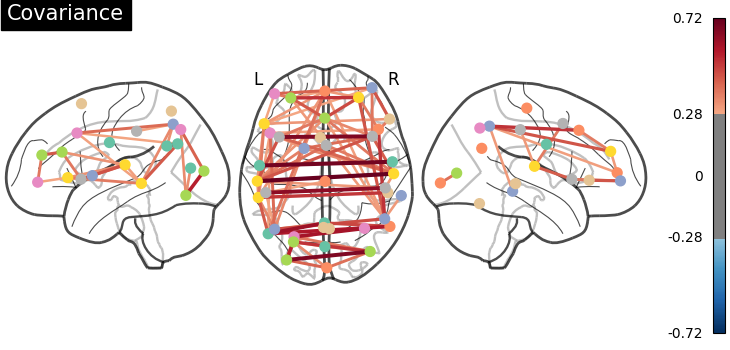•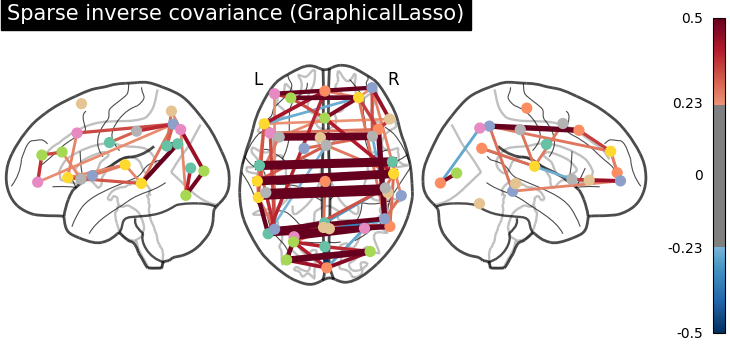•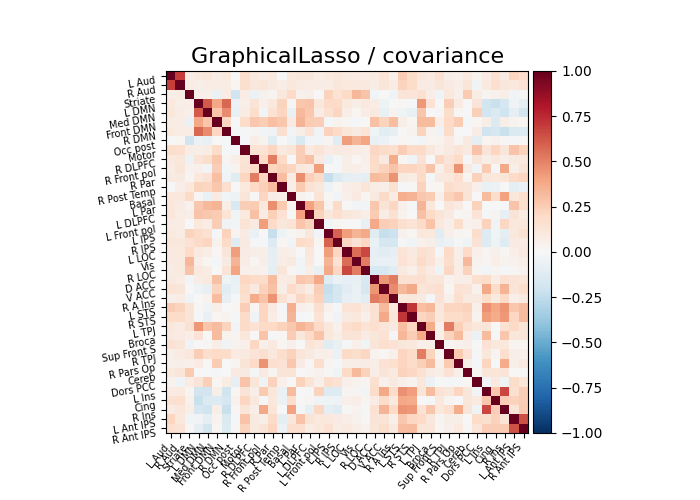•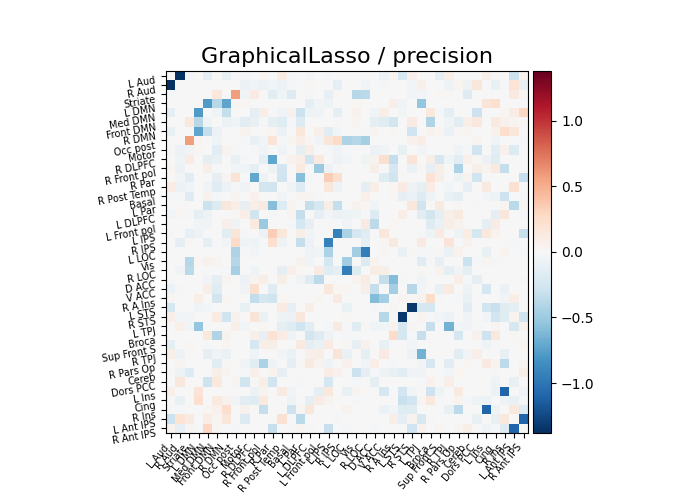•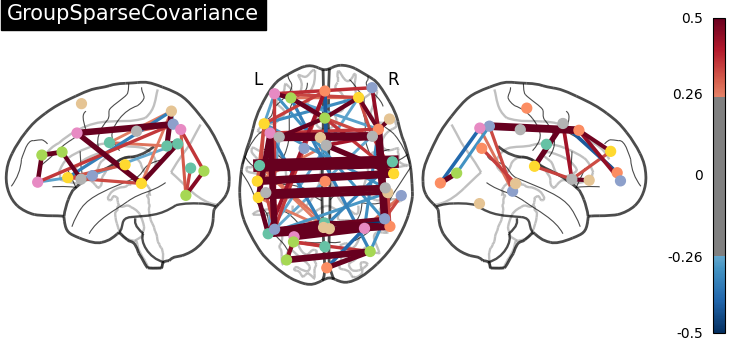•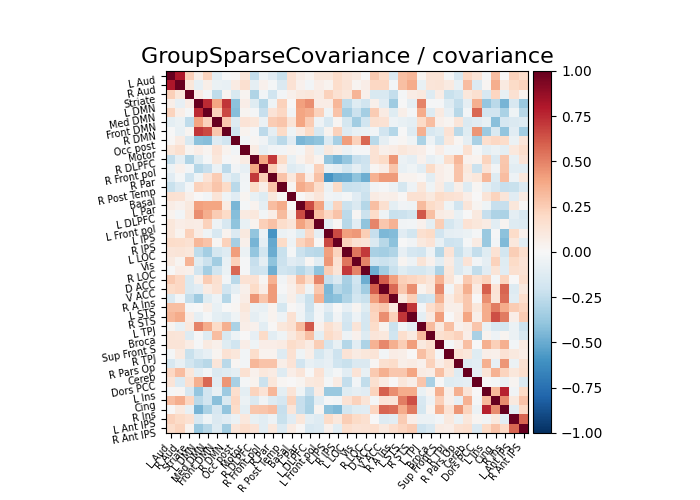•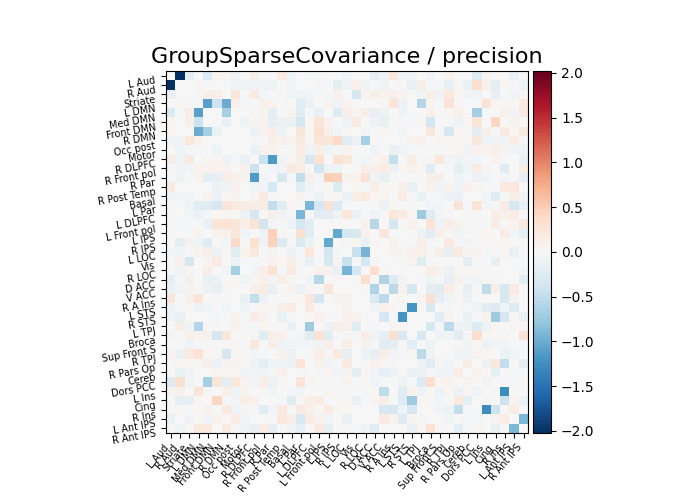Total running time of the script: ( 1 minutes 39.127 seconds)

Estimated memory usage: 610 MB

Gallery generated by Sphinx-Gallery